• 2020-09-24 01:24:15

# xlabel函数使用–Matplotlib

#### xlabel语法、功能与参数设置可平移至ylabel。

函数语法：
xlabel(xlabel, fontdict=None, labelpad=None, *, loc=None, **kwargs)
函数功能：
Set the label for the x-axis.
设置X轴的标签
函数参数：
xlabel： str The label text.
字符串格式，标签文本内容
fontdict： dict, 一个字典用来控制标签的字体样式
labelpad： float, default: None ,Spacing in points from the axes bounding box including ticks and tick labels.
浮点数，默认值为None， x/y轴的标签离x/y轴的偏移量，以点为单位
loc(location): {‘left’, ‘center’, ‘right’}, default: rcParams[“xaxis.labellocation”] (default: ‘center’) The label position. This is a high-level alternative for passing parameters x and horizontalalignment.
标签位置，默认在中间，对于x轴是’left’，‘center’，‘right’，对于y轴则是’bottom’， ‘center’， ‘top’

Other Parameters: **kwargsText properties 。Text properties control the appearance of the label.
其他参数 ： 设置其他文本属性，控制标签文本的展示外观通过loc参数改变y轴标签的位置import matplotlib.pyplot as plt
import numpy as np

x = np.linspace(0.05, 10, 1000)
y = np.cos(x)

plt.plot(x, y,lw = 2,label = 'plot figure')

plt.legend(loc = 'lower left') #指定图例位置

plt.ylabel('testylabel',loc = 'center',
fontdict = {'fontsize' : 15,'fontstyle' : 'oblique',
'color':'red','fontfamily': 'fantasy'})  #设置标签字体字典

plt.show()


font_dict控制字体式样font_dict里面的参数与具体可取值还需要后续在学习发现，目前尚不十分清楚python
更多相关内容
• ## matplotlib中ylabel和xlabel函数

万次阅读 多人点赞 2020-09-22 23:45:48
1.语法 matplotlib.pyplot.ylabel(s, *args, **kwargs) 参数： fontsize:数字或’small’，‘large’，‘medium’ verticalalignment：‘top’, ‘bottom’, ‘center’,...默认：plt.xlabel(fontsize=‘large

1.语法

matplotlib.pyplot.ylabel(s, *args, **kwargs)


参数：
fontsize:数字或’small’，‘large’，‘medium’

verticalalignment：‘top’, ‘bottom’, ‘center’,‘baseline’

horizontalalignment：‘center’, ‘right’, ‘left’

rotation: ‘vertical’,‘horizontal’
默认：plt.xlabel(fontsize=‘large’,verticalalignment=‘top’,horizontalalignment=‘center’，rotation=‘horizontal’)
plt.ylabel(fontsize=‘large’,verticalalignment=‘bottom’,horizontalalignment=‘center’，rotation=‘vertical’)
2.例子:
参数fontsize的值为为数字时可调节字体大小，也可以填写’small’，‘large’，‘medium’，默认为’large’

plt1.xlabel("Value",fontsize='small')
plt2.xlabel("Value",fontsize='medium')
plt3.xlabel("Value",fontsize='large')参数verticalalignment的值为’top’, ‘bottom’, ‘center’,‘baseline’，意思为上下平移向figure与axis之间的中间线对齐，字母底端为‘top’
xlabel与ylabel的默认值不同

plt1.xlabel("Value",fontsize=14,verticalalignment='top')
plt2.xlabel("Value",fontsize=14,verticalalignment='bottom')
plt3.xlabel("Value",fontsize=14,verticalalignment='center')
plt4.xlabel("Value",fontsize=14,verticalalignment='baseline')参数horizontalalignment为’center’, ‘right’, ‘left’ 意思为左右平移向中间对齐.如’left’,若为xlabel则标识最左边对齐figure的中垂线，若为ylabel则标识最左边对齐figure的中横线

plt1.xlabel("Value",fontsize=14,horizontalalignment='center')
plt2.xlabel("Value",fontsize=14,horizontalalignment='right')
plt3.xlabel("Value",fontsize=14,horizontalalignment='left')参数rotation的值为 ‘vertical’,‘horizontal’，意思为旋转

plt1.xlabel("Value",fontsize=14,rotation='vertical')
plt2.ylabel("Value",fontsize=14,rotation='horizontal')旋转后要用到verticalalignment或horizontalalignment时，要注意字母的方向，如

plt.ylabel("Square of Value",
fontsize=14,rotation='horizontal',verticalalignment='top')而此时再用horizontalalignment对准的线变为figure与axis之间的中线,不再为figure的中横线。

plt3.ylabel("Square of Value",
fontsize=14,rotation='horizontal',
verticalalignment='top',horizontalalignment='left')展开全文• 目录 一.语法 1.输入参数 2.名称-值对组参数 3.输出参数 二.说明 三.示例 1.为 x 轴添加标签 ...xlabel函数是为 x 轴添加标签。 一.语法 xlabel(txt) xlabel(target,txt) xlabel.

目录

语法

说明

示例

为 x 轴添加标签

创建多行 x 轴标签

将希腊字母包括在 x 轴标签中

在 x 轴标签中包括变量值

在轴标签中包括上标和下标

更改 x 轴标签字体大小和颜色

为特定绘图的 x 轴添加标签

在创建后修改 x 轴标签

xlabel函数是为 x 轴添加标签

## 语法

xlabel(txt)
xlabel(target,txt)
xlabel(___,Name,Value)
t = xlabel(___)

## 说明

​xlabel(txt) 将为 gca 命令返回的当前坐标区或图的 x 轴添加标签。重新发出 xlabel 命令会将旧标签替换为新标签。

​xlabel(target,txt) 为指定的目标对象添加标签。

xlabel(___,Name,Value) 使用一个或多个名称-值对组参数修改标签外观。

例如，'FontSize',12 将字体大小设置为 12 磅。在所有其他输入参数之后指定名称-值对组参数。并非所有图形类型均支持修改标签外观。

t = xlabel(___) 返回用作 x 轴标签的文本对象。使用 t 可在创建标签后对其进行修改。

## 示例

### 为 x 轴添加标签

将 Population 显示在 x 轴下。

plot((1:10).^2)
xlabel('Population')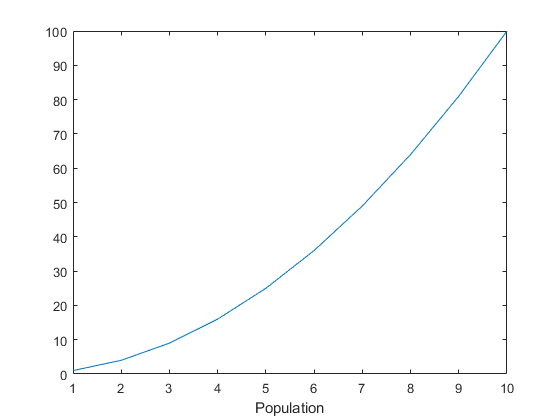### 创建多行 x 轴标签

使用字符向量元胞数组创建多行标签。

plot((1:10).^2)
xlabel({'Population','(in thousands)'})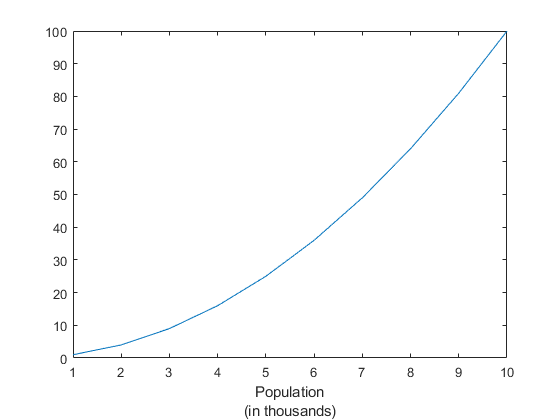### 将希腊字母包括在 x 轴标签中

使用 TeX 标记将希腊字母和其他特殊字符包括在标签中。

x = linspace(-2*pi,2*pi);
y = sin(x);
plot(x,y)
xlabel('-2\pi \leq x \leq 2\pi')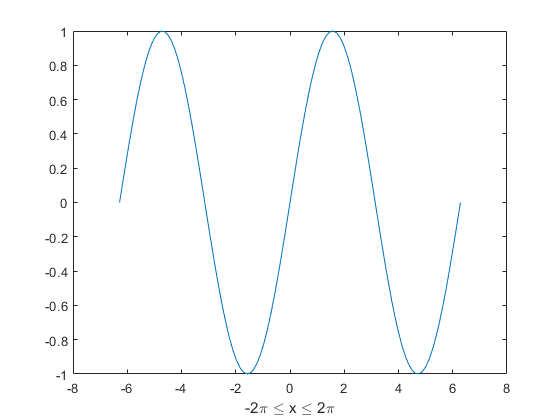### 在 x 轴标签中包括变量值

添加带有文本和变量值的标签。使用 num2str 函数在标签中包含变量值。

plot((1:10).^2)
year = 2014;
xlabel(['Population for Year ',num2str(year)])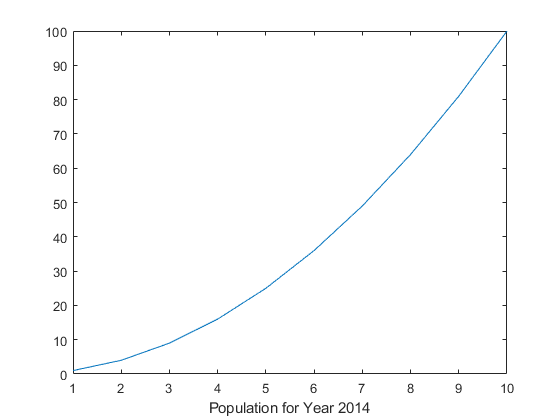### 在轴标签中包括上标和下标

使用 '^' 和 '_' 字符在轴标签中包含上标和下标。使用花括号 {} 修改多个字符。

t = linspace(0,1);
y = exp(t);
plot(t,y)
xlabel('t_{seconds}')
ylabel('e^t')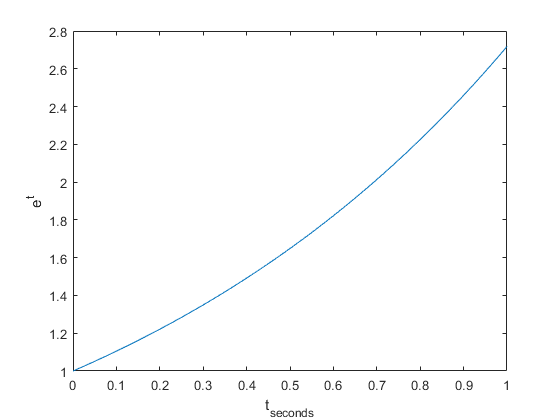### 更改 x 轴标签字体大小和颜色

使用 Name,Value 对组参数设置 x 轴标签的字体大小、字体粗细和文本颜色属性。

plot((1:10).^2)
xlabel('Population','FontSize',12,'FontWeight','bold','Color','r')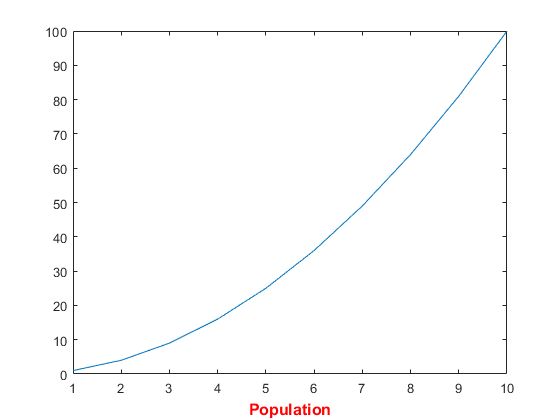### 为特定绘图的 x 轴添加标签

从 R2019b 开始，可以使用 tiledlayout 和 nexttile 函数显示分块图。调用 tiledlayout 函数以创建一个 2×1 分块图布局。调用 nexttile 函数以创建坐标区对象 ax1 和 ax2。将数据绘制到每个坐标区中，并为顶部图创建 x 轴标签。

tiledlayout(2,1)
ax1 = nexttile;
plot((1:10).^2)
xlabel(ax1,'Population')

ax2 = nexttile;
plot((1:10).^3)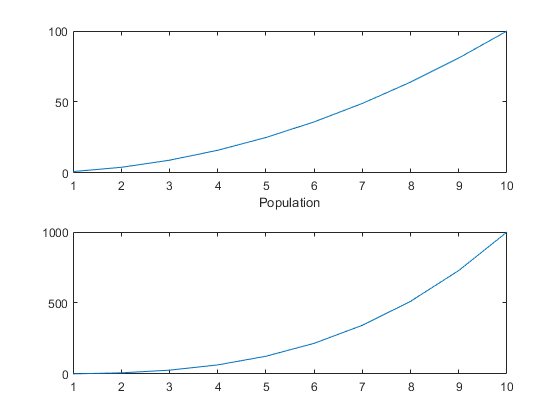### 在创建后修改 x 轴标签

为 x 轴添加标签并返回用作标签的文本对象。

plot((1:10).^2)
t = xlabel('Population');使用 t 可在创建标签后设置标签的文本属性。例如，将标签的颜色设置为红色。使用圆点表示法设置属性。

t.Color = 'red';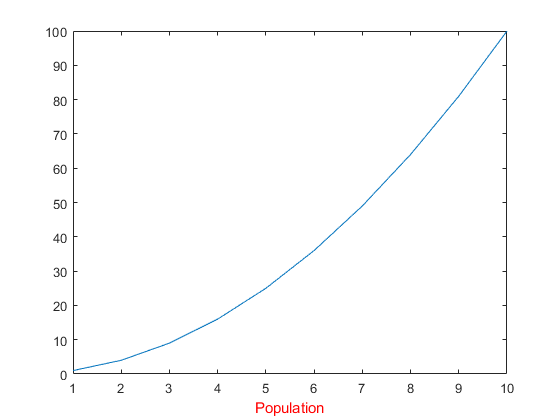展开全文matlab 开发语言
• MATLAB 图形标注与修饰函数 在 MATLAB中，可利用 Figure窗口的菜单和工具栏对图形进行标注、修饰等，操作简单，此外，还可以利用 MATLAB中自带的函数来进行图形的修饰。下面将对其进行介绍 绘制图形以后，需要对图形...

# MATLAB 图形标注与修饰函数

在 MATLAB中，可利用 Figure窗口的菜单和工具栏对图形进行标注、修饰等，操作简单，此外，还可以利用 MATLAB中自带的函数来进行图形的修饰。下面将对其进行介绍
绘制图形以后，需要对图形进行标注、说明等修饰性的处理，以增加图的可读性，使之反映出更多的信息。
在 MATLAB中，可利用 Figure窗口的菜单和工具栏对图形进行标注、修饰等，操作简单，此外，还可以利用 MATLAB中自带的函数来进行图形的修饰。下面将对其进行介绍

### title 函数

title函数用于给当前轴加上标题。每个axes图形对象都可以有一个标题。标题定位于axes的上方正中央。该函数的用法如下title（' string"）：表示在当前坐标轴上方正中央放置字符串 string作为标题

在当前坐标轴上方正中央放置字符串“正弦函数”作为标题。
x=-pi:0.1:pi;
y=sin(x);
plot(x,y)
title('正弦函数')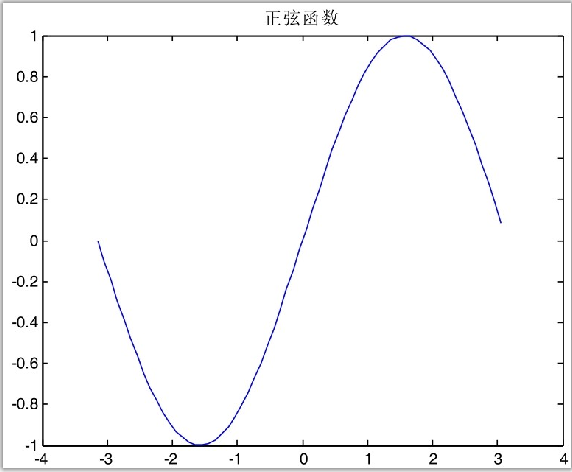### xlabel、ylabel 函数

xlabel、ylabel函数用于给x、y轴贴上标签。该函数的用法如下。
xlabel(‘string’)：表示给当前轴对象中的x轴贴标签。
ylabel(‘string’)：表示给当前轴对象中的y轴贴标签。
利用 xlabel、ylabel、title 对函数图像进行标注。

fplot('sin(x)./x',[-20 20 -0.4 1.2]);
title('Fplot of f(x)=sin(x)/x');
xlabel('x'),
ylabel('f(x)')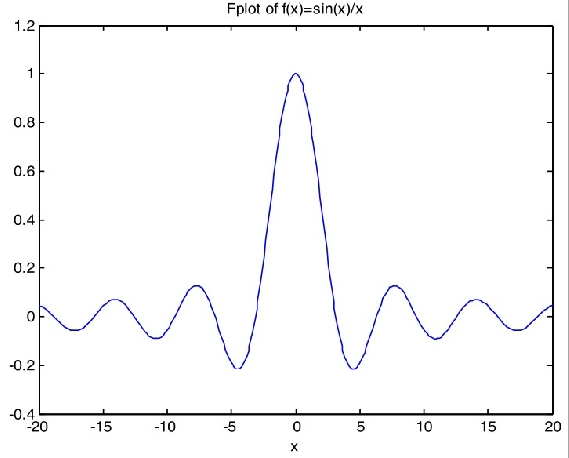### grid 函数

grid函数用于给二维或三维图形的坐标面增加分隔线。该函数的用法如下。
grid on：表示给当前的坐标轴增加分隔线。
grid off：表示从当前的坐标轴中去掉分隔线。
grid：表示转换分隔线的显示与否的状态。

x=-pi:0.1:pi;
y=sin(x);
plot(x,y)
title('正弦函数')
grid on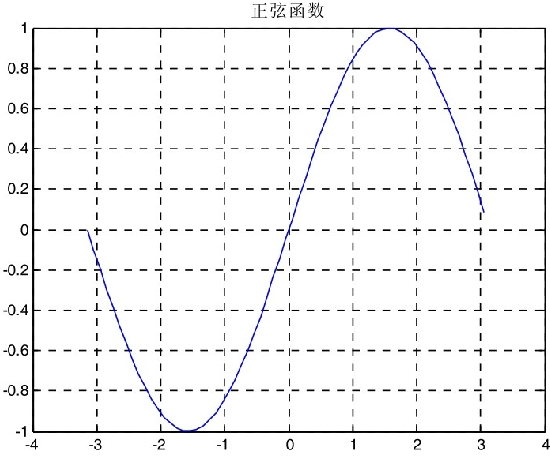### legend 函数

legend函数用于在图形上添加图例。该命令对有多种图形对象类型（线条图、条形图、饼形图等）的窗口显示一个图例。对于每一线条，图例会在用户给定的文字标签旁显示线条的线型、标记符号和颜色等。该函数的用法如下。
legend('string1', 'string2',…, pos)：表示用指定的文字string，在当前坐标轴中对所给数据的每一部分显示一个图例，在指定的位置pos放置这些图例。
使用函数legend在图形中添加图例。

x=magic(3);bar(x);
legend('第一列','第二列','第三列',2);
grid on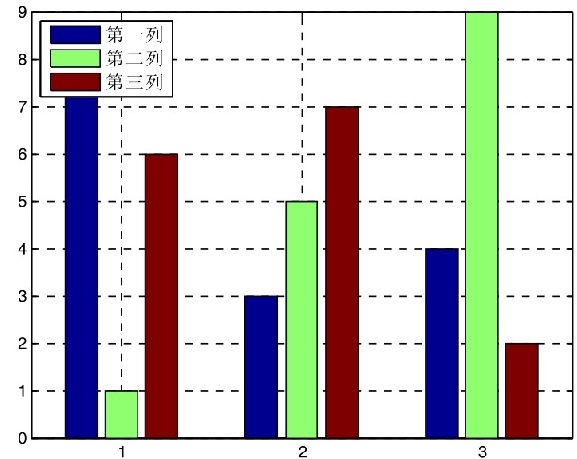### gtext 函数

gtext函数用于在当前二维图形中用鼠标放置文字。当光标进入图形窗口时，会变成一个大十字，表明系统正等待用户的动作。该函数的用法如下。
gtext('string')：表示当光标位于一个图形窗口内时，等待用户单击鼠标或键盘。若按下鼠标或键盘，则在光标的位置放置给定的文字。
使用函数gtext可以将一个字符串放到图形中，位置由鼠标来确定。

plot(peaks(80));
gtext('优美的图形','fontsize',16)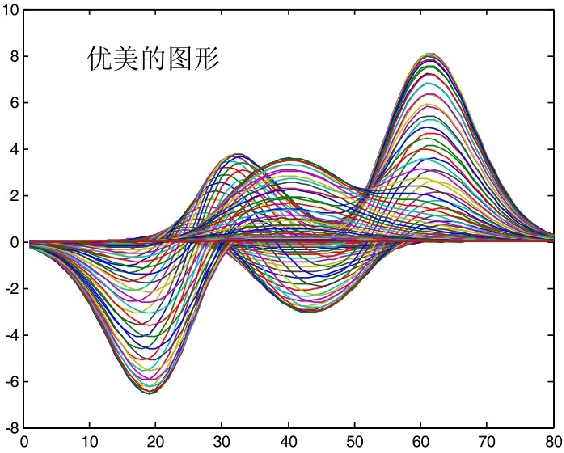### text 函数

text函数用于在当前轴中创建text对象。函数text是创建text图形句柄的低级函数。可用该函数在图形中指定的位置上显示字符串。该函数的用法如下。
text(x,y,'string')：表示在图形中指定的位置(x,y)上显示字符串string。
利用函数text将文本字符串放置在图形中的任意位置。

x=0:pi/100:6;
plot(x,sin(x));
text(3*pi/4,sin(3*pi/4),'\leftarrowsin(x)=0.707','fontsize',14); ％放置文本字符串
text(pi,sin(pi),'\leftarrowsin(x)=0','fontsize',14);
text(5*pi/4,sin(5*pi/4),'sin(x)=-0.707\rightarrow','horizontal','right','fontsize',14);

展开全文matlab
• 调用签名：plt.xlabel(string) string：标签文本内容 平移性：上面的函数功能，也适用于ylabel()上。 代码实现： import matplotlib.pyplot as plt import numpy as np x = np.linspace(0.05, 10, 1000) ...
• Matlab 的 text/ title/ xlabel/ ylabel 函数支持 TeX、LaTeX 排版语法，如希腊字母、上下标、特殊符号等等。 具体流程 调用上述 4 种函数时可以获得相应的句柄，再调用 set 函数向相应句柄设置属性值 txt = xlabel...matlab
• # @File : xlabel.py # @Author: Mr. Luo # @Date: 2021/11/4 13:22 ''' feature:设置x轴的标签文本 ''' # -*- encoding: utf-8 -*- # @File : xlim.py # @Author: Mr. Luo # @Date: 2021/11/4 13:16 # -*- ...python 开发语言 后端
• ## MATLAB xlable的使用

千次阅读 2020-09-08 17:33:32
xlabel('正弦函数') 效果： 多行 xlabel({'正弦函数','sin'}) 特殊字符 xlabel({'正弦函数','sin','-2\pi \leq x \leq 2\pi'}) 改变大小及加粗 xlabel({'正弦函数','sin','-2\pi \leq x \leq 2\...
• 标注x，y和z轴语法xlabel('string')xlabel(fname)xlabel(...,'PropertyName',PropertyValue,...)xlabel(axes_handle,...)h = xlabel(...)ylabel(...)ylabel(axes_handle,...)h = ylabel(...)zlabel(...)zlabel(axes_...
• matplotlib 关于plt.title， plt.xlabel等出现中文乱码问题的解决方案 出现乱码的原因： matplotlib内无中文字节码，需要自行手动添加 解决方案： 导入库函数 from matplotlib.font_manager import FontProperties...
• year)#设置x轴的刻度线为new_year，new_year可以为数组 4、plt.xlabel plt.xlabel('year')#x轴标签 5、plt.plot plt.plot(number, color='blue', label="actual value")#将实际值的折线设置为蓝色 6、两个图分开 fig...
• pyplot.xlabel(u ' 中文 ' , fontproperties= font_set) 7 pyplot.ylabel(u ' 中文 ' , fontproperties=font_set)      结果　 　Successfully ！！！ 　有趣的事，Python永远不会缺席！还不来加...
• 本文介绍了python 设置xlabel,ylabel 坐标轴字体大小，字体类型，分享给大家，具体如下： #--coding:utf-8-- import matplotlib.pyplot as plt #数据设置 x1 =[0,5000,10000, 15000, 20000, 25000, 30000, 35000, ...
• 基于MATLAB的数字图像处理（一）——图像的灰度变换（不直接使用内置函数matlab 计算机视觉
• 如果要输出希腊字母，可以使用texlabel函数将希腊 字母的变量名转化为希腊字母的函数，供函数title、xlabel、ylabel、zlabel或text使用。texlabel转换MATLAB表达式为等价的Tex格式字符串。它处理希腊字母的变量名为...
• 函数与分段函数绘制函数曲线直接使用M文件使用函数 function分段...xlabel(‘x’) ylabel(‘y’) 使用函数 function function[输出变量] = 函数名(输入变量) 改写上面示例 定义函数：function y = myfun( x ) y = x.*
• plt.xlabel('X轴数值') plt.ylabel('y轴数值') plt.title('显示曲线') 但显示出来的图像却。。。 改：在代码上面加入这两行 plt.rcParams['font.family'] = ['sans-serif'] plt.rcParams['font.sans-serif']...python 开发语言
• 如下所示： import numpy as np import matplotlib.pyplot as plt x = np.linspace(0, 2 * ...以上这篇python 画函数曲线示例就是小编分享给大家的全部内容了，希望能给大家一个参考，也希望大家多多支持软件开发网。
• Python matplotlib pyplot中title() xlabel() ylabel()无法显示在中文（方框乱码）的解决办法
• 子图添加序号 1. matlab中subplot子图使用title...2. 使用xlabel函数 3. 使用text函数 Ref: Matlab使用subplot绘制子图时编号（a）（b）©（d） 修改字体 set(gca,'FontName','Times New Roman'); 子图调整边距 ......